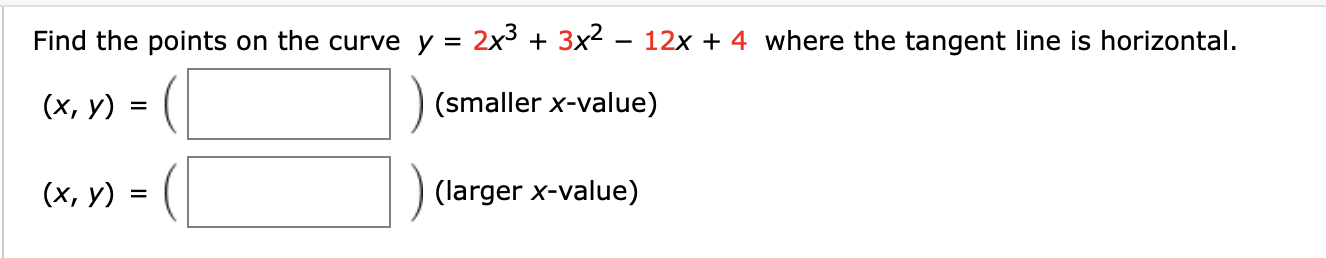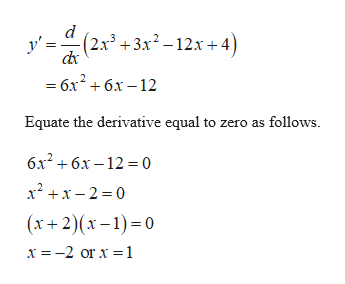Find the points on the curve y = 2x33x2 - 12x4 where the tangent line is horizontal.(х, у)(smaller x-value)(х, у) з(larger x-value)

Questionhelp_outlineImage TranscriptioncloseFind the points on the curve y = 2x3 3x2 - 12x 4 where the tangent line is horizontal. (х, у) (smaller x-value) (х, у) з (larger x-value) fullscreen
Step 1

Find the points on the curve where the tangent line is horizontal as follows.

The given curve is y = 2x3+3x2–12x...help_outlineImage Transcriptionclosed (2х3 + 3х? - 12х + 4) y' — бх* + 6х —12 Equate the derivative equal to zero as follows бх? + бх -12 %3D0 x2x2 0 (х+ 2)(х -1)-0 x -2 or x 1 fullscreen

Want to see the full answer?

See Solution

Want to see this answer and more?

Our solutions are written by experts, many with advanced degrees, and available 24/7

See Solution
Tagged in

Other